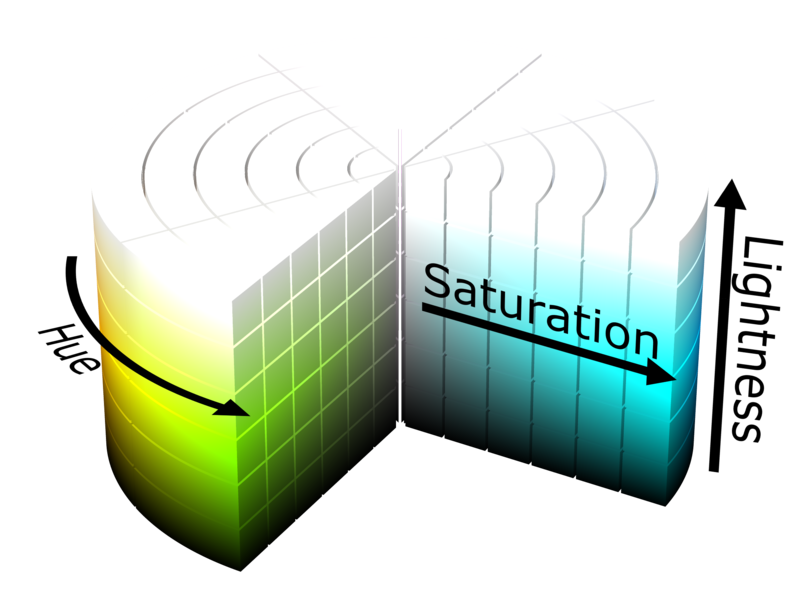Sometimes we need to generate a range of random colors. This can, for example, be when creating graphs where every curve should have a different color to distinguish the curves from each other. A decent first attempt at creating a random-color generator could be a function like this:

``````function randomRgbaString (alpha) {
let r = Math.floor(Math.random() * 255)
let g = Math.floor(Math.random() * 255)
let b = Math.floor(Math.random() * 255)
let a = alpha
return `rgba(\${r},\${g},\${b},\${a})`
}
``````

that is run like this:

``````let colors = [];
for (let i = 0; i < 10; i++) colors.push(randomRgbaString(1));
``````

This generates rgba strings that represents colors in CSS. `alpha` is the alpha-channel, which represents transparency. It’s not important here and is simply set to 1 (no transparency). The color values in the string are random values between 0 and 255, e.g.

``````rgba(127,2,241,1)
rgba(128,54,180,1)
...
rgba(77,149,200,1)
``````

A problem quickly arises when we, for example, generate 10 random colors:

Several of the colors look similar, making it hard to distinguish them from each other. So we don’t really want the colors completely random, we want them spread apart from each other. This is easily done with HSL colors.

### HSL

Hue-Saturation-Lightness (HSL) is an alternative to the RGB color model. Rather than having three values that represents red, green and blue, we now have three values representing hue, saturation and lightness.

HSL has a cylindrical geometry, as seen in the image below.Made by SharkD, under CC BY-SA 3.0.

Hue:

Hue is the perceived color, for example red, green, blue or yellow. The unit of hue is degrees, because the HSL model is represented as a cylinder with hue being the circular coordinate. Red is 0°, green is 120° and blue is 240°.

 0° 40° 80° 120° 160° 200° 240° 280° 320°

The colors above all have constant saturation and lightness.

Saturation:

Saturation represents how “colorful” the color is. It has a value between 0 and 100%. 0% is unsaturated (gray), while 100% is full saturation.

The table below shows various hues with different values of saturation. The saturation increases the further right you go in the table. The lightness is 50% for all the colors.

 Hue / Sat 0% 10% 20% 30% 40% 50% 60% 70% 80% 90% 100% 0° 60° 120° 180° 240° 300°

Lightness:

Lightness is simply the brightness of the color. It has a value between 0 and 100%. 0% lightness is black, while 100% lightness is white. 50% is “normal” colors.

The table below shows various hues with different values of lightness. The lightness increases the further right you go in the table. The saturation is 100% for all the colors.

 Hue / Lig 0% 10% 20% 30% 40% 50% 60% 70% 80% 90% 100% 0° 60° 120° 180° 240° 300°

### Code

Using this information we can create a function that generates colors as `hsla()` strings, which are also supported by CSS. The strings should be returned in an array and the colors should not be similar to each other.

The perceived color is basically the hue, which means we can keep the saturation and lightness constant. The number of colors we want to generate is `amount`. By dividing 360° by `amount` we get the difference between the hues of the returned colors.

E.g. `amount = 2` gives the hues 0° and 180°. `amount = 3` gives the hues 0°, 120° and 240°.

Saturation and lightness are in percent, while alpha is between 0 and 1.

``````function generateHslaColors (saturation, lightness, alpha, amount) {
let colors = []
let huedelta = Math.trunc(360 / amount)

for (let i = 0; i < amount; i++) {
let hue = i * huedelta
colors.push(`hsla(\${hue},\${saturation}%,\${lightness}%,\${alpha})`)
}

return colors
}
``````

### Examples

``````let c = generateHslaColors(50, 100, 1.0, 3)
``````
 c c c

``````let c = generateHslaColors(50, 100, 1.0, 5)
``````
 c c c c c

``````let c = generateHslaColors(50, 100, 1.0, 7)
``````
 c c c c c c c

``````let c = generateHslaColors(50, 100, 1.0, 9)
``````
 c c c c c c c c c

We can see that we get colors spread all over the color spectrum, rather than having several colors that look similar. The function won’t work very well when we are asking for a large amount of colors. If we ask for 36 colors, for instance, we only have a hue delta of 10°. The colors are then barely distinguishable. For cases like that the saturation and lightness also have to vary.